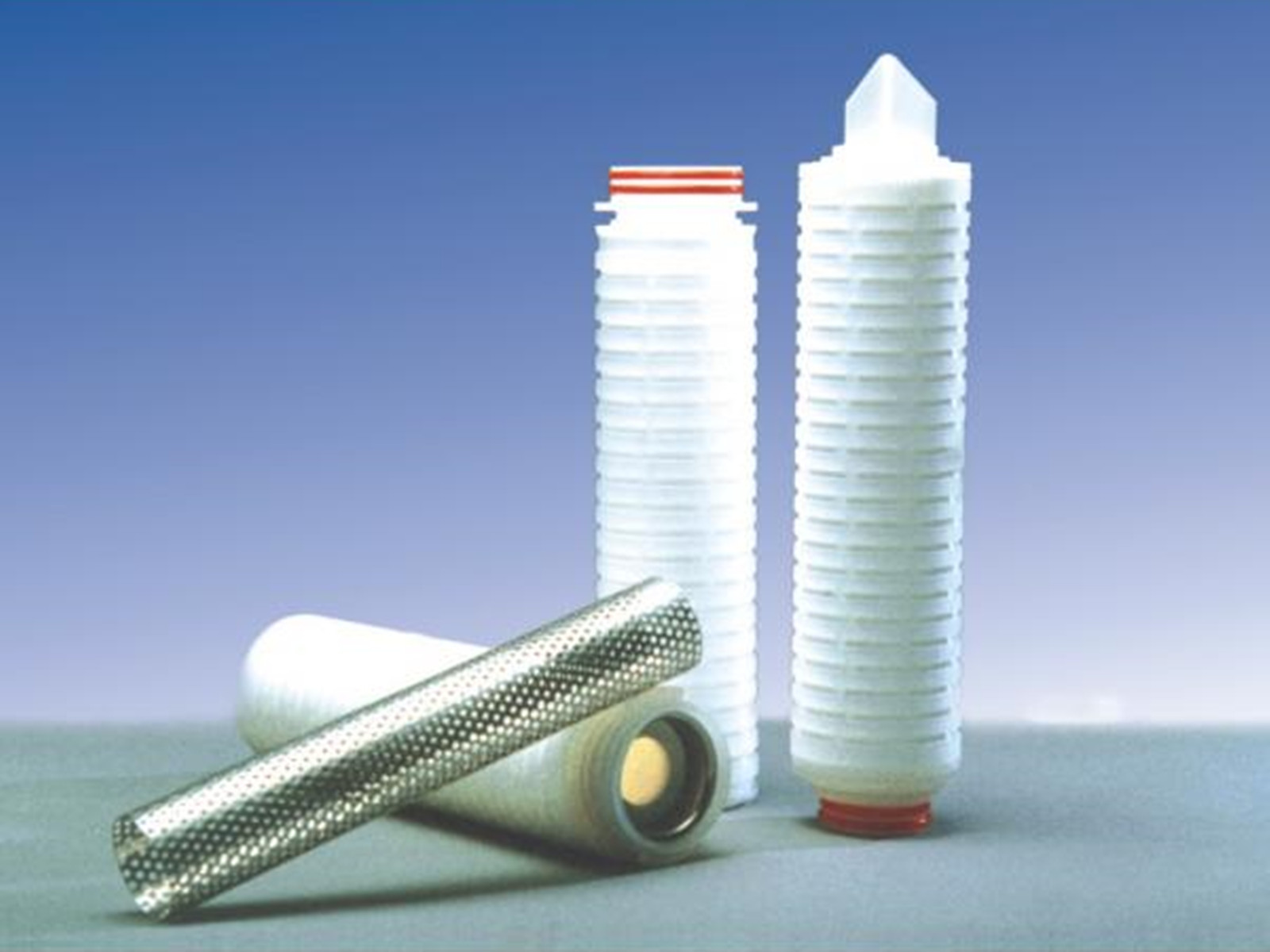# 公司产品# GF玻璃纤维膜折叠滤芯

LGF系列折叠滤芯采用超细玻璃纤维材料制成，具有90%以上的容污空间和固有的吸附作用，尤其适合过滤含有胶体、油脂以及颗粒含量较高的料液。起始压差低，流量大，使用寿命长。可达到98%以上极高的截留效率。

• 产品概述
• 规格参数
• 产品选型

GF玻璃纤维膜折叠滤芯

LGF系列折叠滤芯采用超细玻璃纤维材料制成，具有90%以上的容污空间和固有的吸附作用，尤其适合过滤含有胶体、油脂以及颗粒含量较高的料液。起始压差低，流量大，使用寿命长。可达到98%以上极高的截留效率。

 酸碱 R 苯 R 乙醇 R 油 R 氢氧化钾 NR 丙酮 R 乙脂乙酸 R 乙醚 R

R: 推荐     NR: 不推荐

0.22、0.45、1.00、3.0、5.0、10

 LGF05H0022M LGF 长度 接口 精度 密封材质 05=5英寸 A=平压 G=222衬钢/翅片 0022=0.22μm S=硅橡胶 10=10英寸 B=222/翅片 H=226衬钢/翅片 0045=0.45μm M=三元乙丙 20=20英寸 C=226/翅片 K=222衬钢/平封 0100=1μm N=丁腈橡胶 30=30英寸 D=222/平封 M=226衬钢/平封 0300=3μm V=氟橡胶 40=40英寸 E=226/平封 0500=5μm T=铁氟龙包氟 F=215/平封 1000=10μm

# 相关推荐X